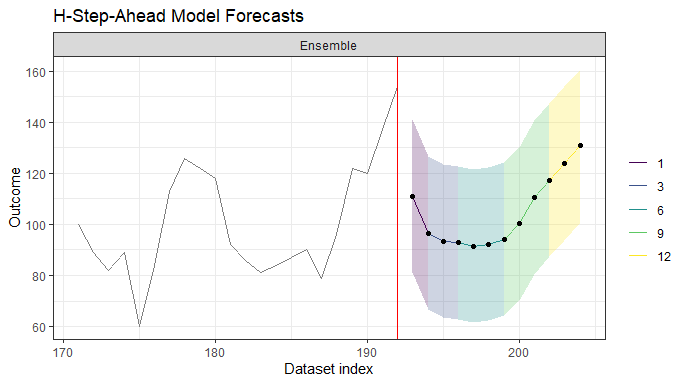# Purpose

The purpose of this vignette is to illustrate the various approaches in forecsatML for producing final forecasts that are (a) a combination of short- and long-term forecasts as well as (b) a combination of many ML models at select forecast horizons.

The goal of forecastML::combine_forecasts() is to provide maximum flexibility when producing a single forecast that is expected to perform as well in the near-term as it is in the long-term.

# Forecast Combination by Horizon

• Forecast combinations with forecastML::combine_forecasts(..., type = "horizon") are a simple and effective method for producing final forecasts that consist of (a) an ensemble of short- and long-term forecasts and (b) an ensemble of separately trained ML models at any forecast horizon.

• Below are 3 examples:

• 1: An ensemble of short- and long-term forecasts
• 2: An ensemble of short- and long-term forecasts with separately trained ML models
• 3: An ensemble of short- and long-term forecasts with a cross-sectional ensemble of models at select horizons

# library(forecastML)
library(dplyr)
library(ggplot2)
library(glmnet)

data("data_seatbelts", package = "forecastML")
data <- data_seatbelts

## 1: One Model Training Function

• Setup:
• 1 model training function (could consist of an ensemble of models).
• Multiple direct forecast horizons.

• Combination:
• Greedy: Models with shorter direct forecast horizons produce near-term forecasts, and models with longer direct forecast horizons only produce forecasts at horizons above and beyond those from the short-term models.

• Pros:
• Easy to implement.

• Cons:
• If the model training function uses 1 ML algorithm–e.g., a neural network–to build both short- and long-term direct forecast models–which will have different parameters/hyperparameters–, it could be the case that entirely different model classes–e.g., support vector machines–may produce better short- or long-term final forecasts if included in the forecast combination.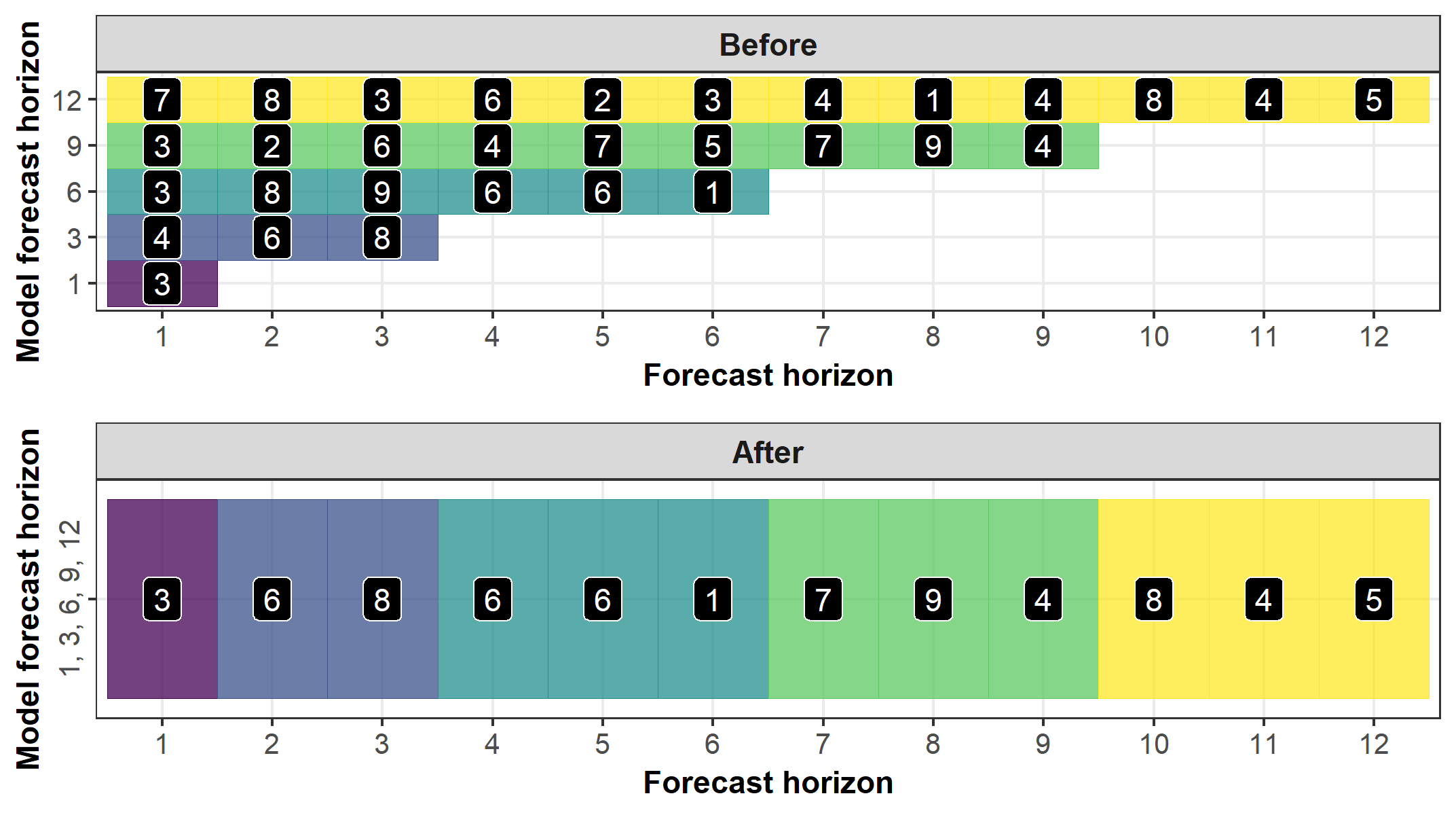• Example:
horizons <- c(1, 3, 6, 9, 12)
data_train <- forecastML::create_lagged_df(data_seatbelts, type = "train", method = "direct",
outcome_col = 1, lookback = 1:15, horizon = horizons)

windows <- forecastML::create_windows(data_train, window_length = 0)

model_fun <- function(data) {
x <- as.matrix(data[, -1, drop = FALSE])
y <- as.matrix(data[, 1, drop = FALSE])
set.seed(1)
model <- glmnet::cv.glmnet(x, y, nfolds = 5)
}

model_results <- forecastML::train_model(data_train, windows, model_name = "LASSO", model_function = model_fun)

prediction_fun <- function(model, data_features) {
data_pred <- data.frame("y_pred" = predict(model, as.matrix(data_features)),
"y_pred_lower" = predict(model, as.matrix(data_features)) - 30,
"y_pred_upper" = predict(model, as.matrix(data_features)) + 30)
}

data_forecast <- forecastML::create_lagged_df(data_seatbelts, type = "forecast", method = "direct",
outcome_col = 1, lookback = 1:15, horizon = horizons)

data_forecasts <- predict(model_results, prediction_function = list(prediction_fun), data = data_forecast)

data_forecasts <- forecastML::combine_forecasts(data_forecasts, type = "horizon")

plot(data_forecasts, data_actual = data_seatbelts[-(1:170), ], actual_indices = (1:nrow(data_seatbelts))[-(1:170)])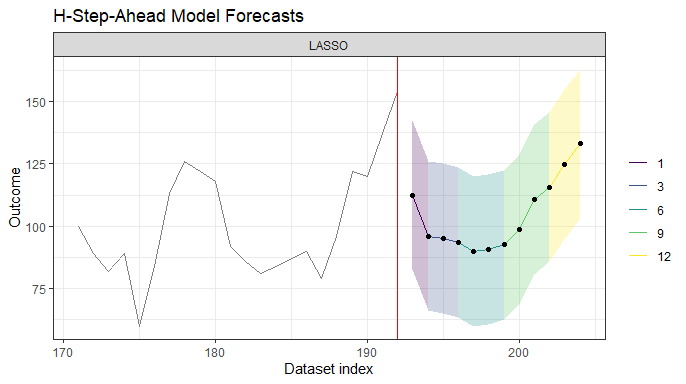## 2: Multiple Model Training Functions

• Setup:
• 2 or more model training functions.
• Multiple direct forecast horizons.

• Combination:
• Greedy: Models with shorter direct forecast horizons produce near-term forecasts, and models with longer direct forecast horizons only produce forecasts at horizons above and beyond those from the short-term models.
• If multiple model training functions produce models with the same direct forecast horizon, forecasts for the shared horizon(s) are combined with the function passed in combine_forecasts(..., agregate = function) (see example 3 below).

• Pros:
• Gives precise control over the algorithms used to produce short- and long-term forecasts. For example, a large neural network may produce the best short-term forecasts while a linear model may produce the best long-term forecasts.
• Also useful with large data when training multiple direct forecast models with one call to forecastML::train_model() uses too much memory. Here, you would train one model at a time and combine them with forecastML::combine_forecasts().

• Cons:
• More code.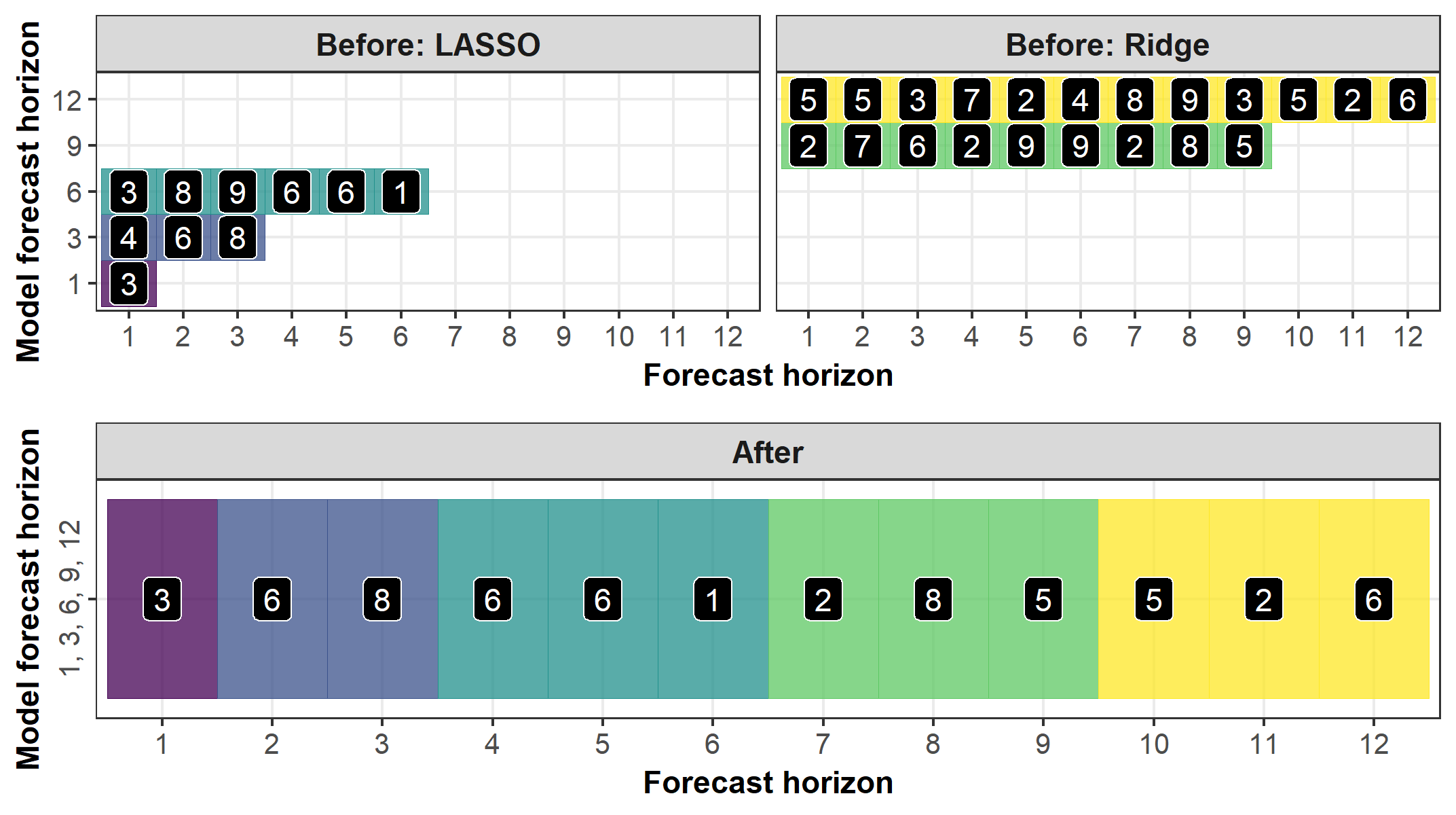• Example:
# LASSO

horizons <- c(1, 3, 6)
data_train <- forecastML::create_lagged_df(data_seatbelts, type = "train", method = "direct",
outcome_col = 1, lookback = 1:15, horizon = horizons)

windows <- forecastML::create_windows(data_train, window_length = 0)

model_fun_lasso <- function(data) {
x <- as.matrix(data[, -1, drop = FALSE])
y <- as.matrix(data[, 1, drop = FALSE])
set.seed(1)
model <- glmnet::cv.glmnet(x, y, alpha = 1, nfolds = 5)
}

model_results <- forecastML::train_model(data_train, windows, model_name = "LASSO", model_function = model_fun_lasso)

prediction_fun <- function(model, data_features) {
data_pred <- data.frame("y_pred" = predict(model, as.matrix(data_features)),
"y_pred_lower" = predict(model, as.matrix(data_features)) - 30,
"y_pred_upper" = predict(model, as.matrix(data_features)) + 30)
}

data_forecast <- forecastML::create_lagged_df(data_seatbelts, type = "forecast", method = "direct",
outcome_col = 1, lookback = 1:15, horizon = horizons)

data_forecasts_lasso <- predict(model_results, prediction_function = list(prediction_fun), data = data_forecast)
#------------------------------------------------------------------------------
# Ridge

horizons <- c(9, 12)
data_train <- forecastML::create_lagged_df(data_seatbelts, type = "train", method = "direct",
outcome_col = 1, lookback = 1:15, horizon = horizons)

windows <- forecastML::create_windows(data_train, window_length = 0)

model_fun_ridge <- function(data) {
x <- as.matrix(data[, -1, drop = FALSE])
y <- as.matrix(data[, 1, drop = FALSE])
set.seed(1)
model <- glmnet::cv.glmnet(x, y, alpha = 0, nfolds = 5)
}

model_results <- forecastML::train_model(data_train, windows, model_name = "Ridge", model_function = model_fun_ridge)

prediction_fun <- function(model, data_features) {
data_pred <- data.frame("y_pred" = predict(model, as.matrix(data_features)),
"y_pred_lower" = predict(model, as.matrix(data_features)) - 30,
"y_pred_upper" = predict(model, as.matrix(data_features)) + 30)
}

data_forecast <- forecastML::create_lagged_df(data_seatbelts, type = "forecast", method = "direct",
outcome_col = 1, lookback = 1:15, horizon = horizons)

data_forecasts_ridge <- predict(model_results, prediction_function = list(prediction_fun), data = data_forecast)
#------------------------------------------------------------------------------
# Forecast combination.

data_forecasts <- forecastML::combine_forecasts(data_forecasts_lasso, data_forecasts_ridge, type = "horizon")

plot(data_forecasts, data_actual = data_seatbelts[-(1:170), ], actual_indices = (1:nrow(data_seatbelts))[-(1:170)])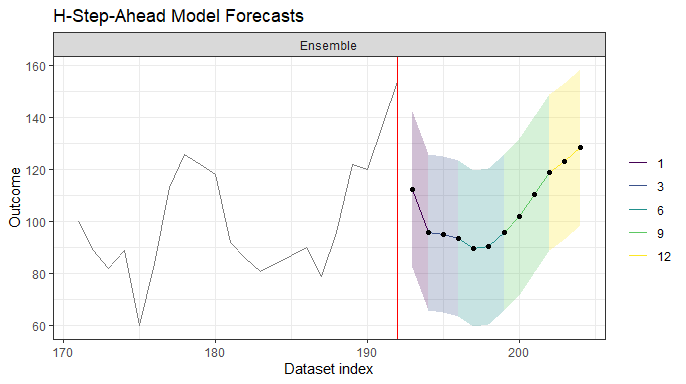## 3: Multiple Model Training Functions - Aggregation

• Setup:
• 2 or more model training functions.
• The model training functions share the same direct forecast horizons (complete overlap is not necessary).
• Multiple direct forecast horizons.

• Combination:
• If multiple model training functions produce models with the same direct forecast horizon, forecasts for the shared horizon(s) are combined with the function passed in combine_forecasts(..., agregate = function).
• The default combination for shared horizons is median().
• Greedy: Models with shorter direct forecast horizons produce near-term forecasts, and models with longer direct forecast horizons only produce forecasts at horizons above and beyond those from the short-term models.

• Pros:
• A simple way to produce ensemble forecasts at a given forecast horizon.

• Cons:
• More code.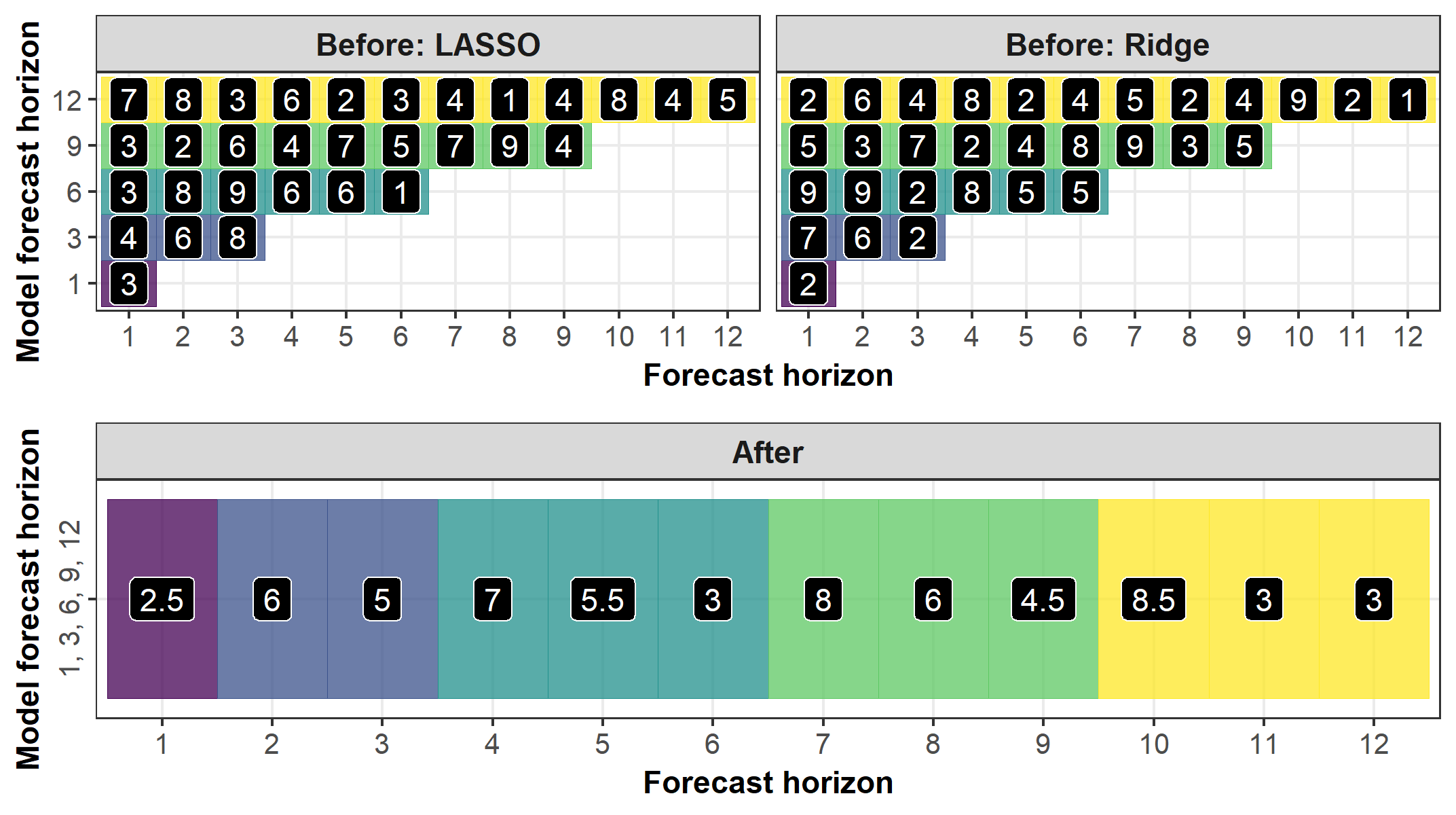• Example:
# LASSO

horizons <- c(1, 3, 6, 9, 12)
data_train <- forecastML::create_lagged_df(data_seatbelts, type = "train", method = "direct",
outcome_col = 1, lookback = 1:15, horizon = horizons)

windows <- forecastML::create_windows(data_train, window_length = 0)

model_fun_lasso <- function(data) {
x <- as.matrix(data[, -1, drop = FALSE])
y <- as.matrix(data[, 1, drop = FALSE])
set.seed(1)
model <- glmnet::cv.glmnet(x, y, alpha = 1, nfolds = 5)
}

model_results <- forecastML::train_model(data_train, windows, model_name = "LASSO", model_function = model_fun_lasso)

prediction_fun <- function(model, data_features) {
data_pred <- data.frame("y_pred" = predict(model, as.matrix(data_features)),
"y_pred_lower" = predict(model, as.matrix(data_features)) - 30,
"y_pred_upper" = predict(model, as.matrix(data_features)) + 30)
}

data_forecast <- forecastML::create_lagged_df(data_seatbelts, type = "forecast", method = "direct",
outcome_col = 1, lookback = 1:15, horizon = horizons)

data_forecasts_lasso <- predict(model_results, prediction_function = list(prediction_fun), data = data_forecast)
#------------------------------------------------------------------------------
# Ridge

horizons <- c(1, 3, 6, 9, 12)
data_train <- forecastML::create_lagged_df(data_seatbelts, type = "train", method = "direct",
outcome_col = 1, lookback = 1:15, horizon = horizons)

windows <- forecastML::create_windows(data_train, window_length = 0)

model_fun_ridge <- function(data) {
x <- as.matrix(data[, -1, drop = FALSE])
y <- as.matrix(data[, 1, drop = FALSE])
set.seed(1)
model <- glmnet::cv.glmnet(x, y, alpha = 0, nfolds = 5)
}

model_results <- forecastML::train_model(data_train, windows, model_name = "Ridge", model_function = model_fun_ridge)

prediction_fun <- function(model, data_features) {
data_pred <- data.frame("y_pred" = predict(model, as.matrix(data_features)),
"y_pred_lower" = predict(model, as.matrix(data_features)) - 30,
"y_pred_upper" = predict(model, as.matrix(data_features)) + 30)
}

data_forecast <- forecastML::create_lagged_df(data_seatbelts, type = "forecast", method = "direct",
outcome_col = 1, lookback = 1:15, horizon = horizons)

data_forecasts_ridge <- predict(model_results, prediction_function = list(prediction_fun), data = data_forecast)
#------------------------------------------------------------------------------
# Forecast combination.

data_forecasts <- forecastML::combine_forecasts(data_forecasts_lasso, data_forecasts_ridge,
type = "horizon", aggregate = stats::median)

plot(data_forecasts, data_actual = data_seatbelts[-(1:170), ], actual_indices = (1:nrow(data_seatbelts))[-(1:170)])